### Home > PC > Chapter 1 > Lesson 1.2.2 > Problem1-85

1-85.
1. Simplify each expression to a single integer with an integral power, or state “impossible” and explain why. Homework Help ✎

1. 23 · 32

2. 22 + 23

3. 22 · 23

4. (22)3

5.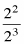6. 22 − 24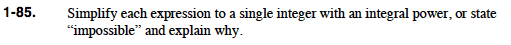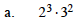Impossible, different bases.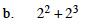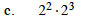$2^{2+3} = 2^5$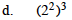$2^{2\cdot 3} = 2^{6}$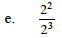$2^{2-3} = 2^{-1}$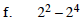Impossible, bases are being subtracted.